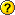# 2. Systems of linear equations and matrices

A system of linear equations is a collection of linear equations involving the same set of variables.

For example,

$$\begin{cases} 5 x_1 + 2 x_2 = 4\\ 3 x_1 + 6 x_2 = 7 \end{cases}$$

is a system of two linear equations, both of then involving two variables $x_1$ and $x_2$ (the unknowns). The numbers $\ 5,\ 2$ and $\ 3,\ 6$ are called the coefficients of the system, and $\ 4$ and $\ 7$ are the constant terms.

A general system of $m$ linear equations with $n$ unknowns can be written as

 $(\ast)$ \begin{align*} \left\{ \begin{aligned} a_{11}x_1 +\, a_{12}x_2\, +\, \dots\, +\, a_{1n}x_n&=b_1\\ a_{21}x_1 +\, a_{22}x_2\, +\, \dots\, +\, a_{2n}x_n&=b_1\\ \dots\qquad\qquad\qquad\qquad&\\ a_{m1}x_1 + a_{m2}x_2 + \dots + a_{mn}x_n&=b_m\\ \end{aligned} \right. \end{align*},

where $\ x_1,\ x_2,\ \dots\ ,\ x_n$ are the unknowns, $\ a_{11},\ a_{12},\ \dots\ ,\ a_{mn}$ are the coefficients of the system, and $\ b_1,\ b_2,\ \dots\ ,\ b_m$ are the constant terms. According to matrix multiplication

$$\begin{pmatrix} a_{11}x_1 +\, a_{12}x_2\, +\, \dots\, +\, a_{1n}x_n\\ a_{21}x_1 +\, a_{22}x_2\, +\, \dots\, +\, a_{2n}x_n\\ \vdots\\ a_{m1}x_1 + a_{m2}x_2 + \dots + a_{mn}x_n \end{pmatrix}= \begin{pmatrix} a_{11}&a_{12}&\dots &a_{1n}\\ a_{21}&a_{22}&\dots &a_{2n}\\ \vdots & \vdots &\ddots&\vdots\\ a_{m1}&a_{m2}&\dots &a_{mn} \end{pmatrix} \begin{pmatrix} x_1\\ x_2\\ \vdots\\ x_m \end{pmatrix}.$$

Since two matrices are equal if and only if all the corresponding elements of the two matrices are equal, and we have

$$a_{11}x_1 +\, a_{12}x_2\, +\, \dots\, +\, a_{1n}x_n=b_1,\\ a_{21}x_1 +\, a_{22}x_2\, +\, \dots\, +\, a_{2n}x_n=b_2,\\ \dots\\ a_{m1}x_1 + a_{m2}x_2 + \dots + a_{mn}x_n=b_m,$$

we can write down the system $(\ast)$ in matrix notation as follows

 $(\ast\ast)$ $$\begin{pmatrix} a_{11}&a_{12}&\dots &a_{1n}\\ a_{21}&a_{22}&\dots &a_{2n}\\ \vdots & \vdots &\ddots&\vdots\\ a_{m1}&a_{m2}&\dots &a_{mn} \end{pmatrix} \begin{pmatrix} x_1\\ x_2\\ \vdots\\ x_m \end{pmatrix}= \begin{pmatrix} b_1\\ b_2\\ \vdots\\ b_m \end{pmatrix}.$$What is the matrix notation for

 $\begin{cases}2 x_1 - 3x_2 = 1\\-7 x_1 + 6 x_2 = 8\end{cases}$ $\begin{cases} -6x_1 +2x_3 = -1\\4x_1 -5x_2 +9 x_3 = 7\\-8x_2 -x_3 = -6 \end{cases}$ $\begin{cases}5x +7y-2x = 3\\-1x-4y+6x = 9\end{cases}$ Answer $\begin{pmatrix}2&-3\\-7&6\end{pmatrix}\begin{pmatrix}x_1\\x_2\end{pmatrix}=\begin{pmatrix}1\\8\end{pmatrix}$ Answer $\begin{pmatrix}-6&0&2\\4&-5&9\\0&-8&-1\end{pmatrix}\begin{pmatrix}x_1\\x_2\\x_3\end{pmatrix}=\begin{pmatrix}-1\\7\\6\end{pmatrix}$ Answer $\begin{pmatrix}3&7\\5&-4\end{pmatrix}\begin{pmatrix}x\\y\end{pmatrix}=\begin{pmatrix}3\\9\end{pmatrix}$

Also, the augmented matrix

$$\left(\begin{array}{rrrr|r} a_{11}&a_{12}&\dots &a_{1n}&b_1\\ a_{21}&a_{22}&\dots &a_{2n}&b_2\\ \vdots & \vdots &\ddots&\vdots&\vdots\\ a_{m1}&a_{m2}&\dots &a_{mn}&b_m \end{array}\right),$$

where the firts row represence the first equation, the second row represence the second equation and so on, has exactly the same information as the original system $(\ast\ast)$. The augmented matrix can be used for solving the system of linear equations.

For example, let us consider solving the system

\begin{align*} \left\{ \begin{aligned} 1x - 2y &= 2\\ 2x + 4y &= 12 \end{aligned} \right. \end{align*}

by using the facts that

• we can multiply an equation (that is, every term of an equation) with a nonzero constant without changing the solution, e.g.,

$5x+3=-2\quad \Longleftrightarrow \quad\color{blue}{\frac{1}{5}}\cdot5x+\color{blue}{\frac{1}{5}}\cdot3=\color{blue}{\frac{1}{5}}\cdot(-2)$;

• an equation which is obtained by adding some value to both sides of an initial equation, has the same solution as the initial equation, e.g.

$5x+3=-2\quad \Longleftrightarrow \quad 5x+3\color{blue}{-3}=-2\color{blue}{-3}$,

but also $\quad 2x+y=7\quad \Longleftrightarrow \quad 2x+y\color{blue}{+4x-y}=7\color{blue}{-2}\text{,}\quad$ if $\quad 4x-y=-2$.

Thus, a step-by-step solution for the system is

\begin{align*} \left\{ \begin{array}{rrrrr} 1x & - & 2y & = & 2\\ 2x & + & 4y & = & 12 \end{array} \right. \overset{R_2-2\cdot R_1}\Longleftrightarrow \left\{ \begin{array}{rrrrr} 1x & - & 2y & = & 2\\ & + & 8y & = & 8\\ \end{array} \right. \overset{\frac{1}{8}\cdot R_2}\Longleftrightarrow \left\{ \begin{array}{rrrrr} 1x & - & 2y & = & 2\\ & & y & = & 1\\ \end{array} \right. \overset{R_1+2\cdot R_2}\Longleftrightarrow \left\{ \begin{array}{rrrrr} x & & & = & 4\\ & & y & = & 1\\ \end{array} \right. \end{align*},

where $R_1$ denotes the first equation and $R_2$ denotes the second equation. It can be written down also in terms of matrices:

\begin{align*} \left(\begin{array}{rr|r} 1 &-2 &2\\ 2 & 4 &12\\ \end{array}\right) \quad\overset{R_2-2\cdot R_1}\longrightarrow\quad \left(\begin{array}{rr|r} 1 &-2 &2\\ 0 & 8 &8\\ \end{array}\right) \quad\overset{\frac{1}{8}\cdot R_2}\longrightarrow\quad \left(\begin{array}{rr|r} 1 &-2 &2\\ 0 & 1 &1\\ \end{array}\right) \quad\overset{R_1+2\cdot R_2}\longrightarrow\quad \left(\begin{array}{rr|r} 1 & 0 &4\\ 0 & 1 &1\\ \end{array}\right)\enspace, \end{align*}

where the first row of the matrices re-presence the first equation and the second row of the matrices re-presence the second equation.

Thus, solving a system of linear equation can be viewed as manipulating the corresponding augmented matrix rows. It is something a computer can easily do and thus, it makes possible to solve a system of linear equations by a computer.

The algorithm of making matrix row manipulations for solving the system of linear equations is known as Gaussian elimination algorithm.